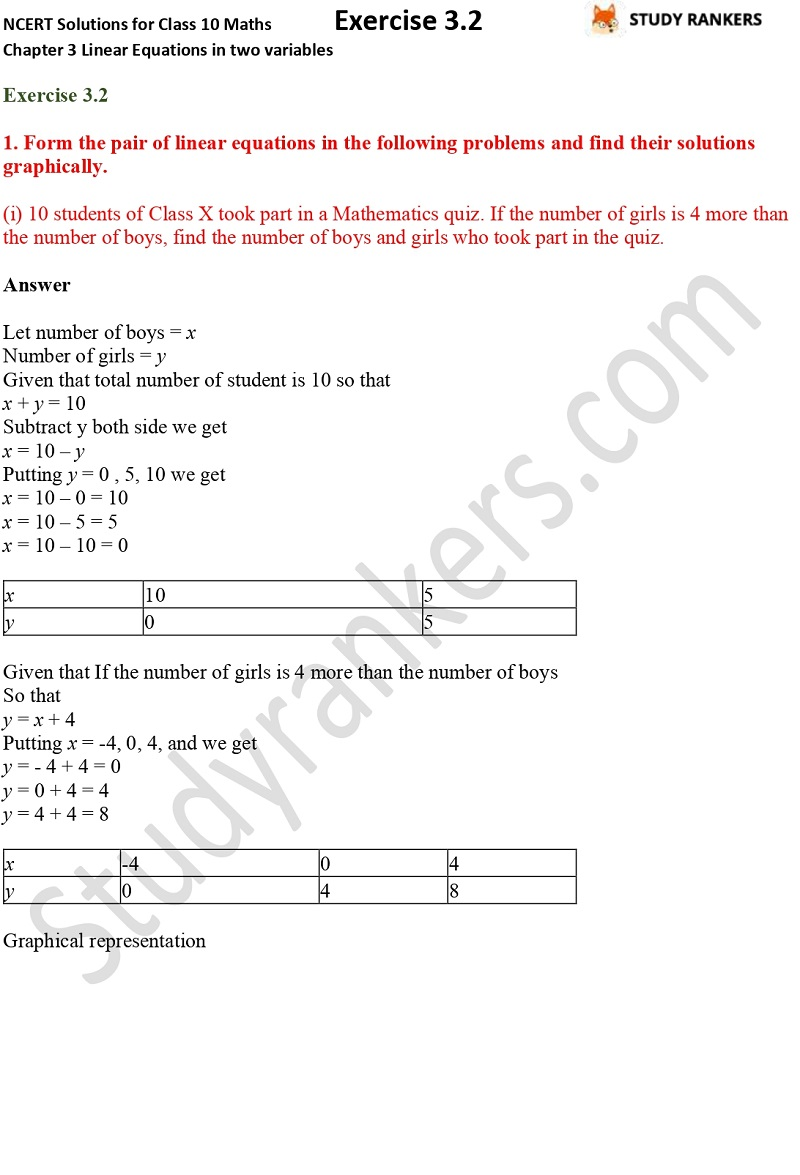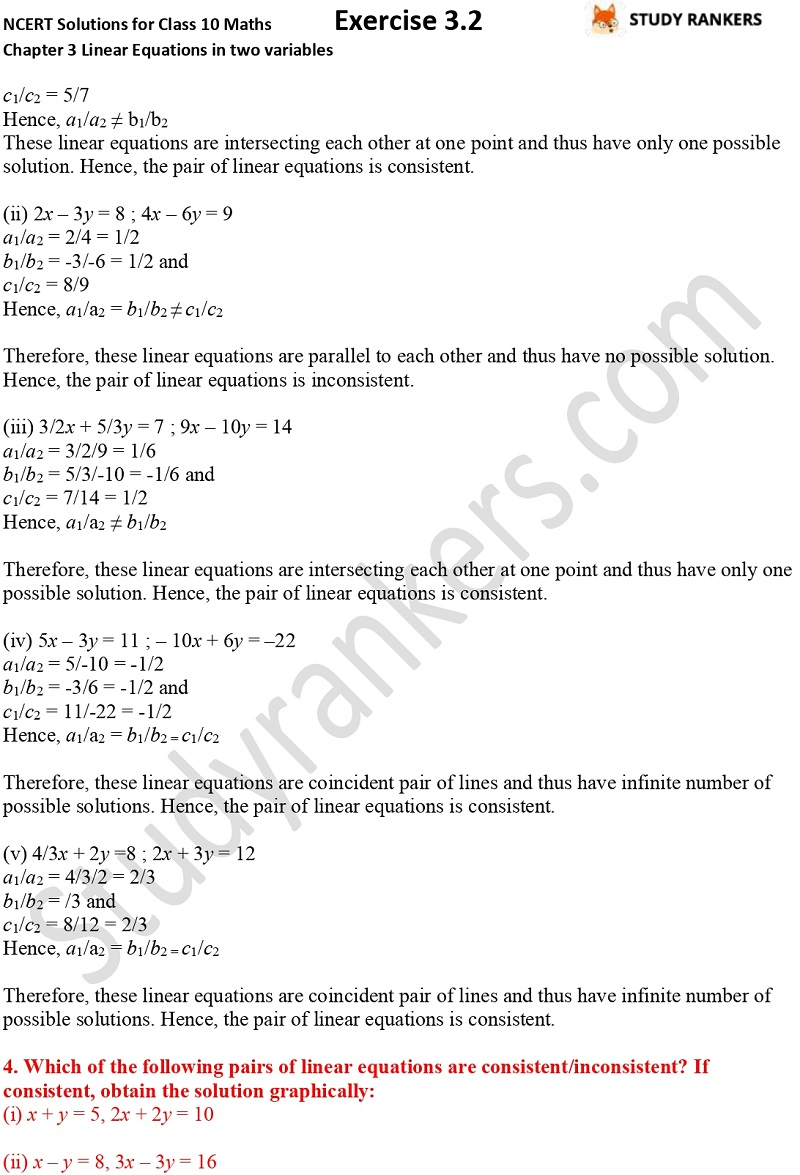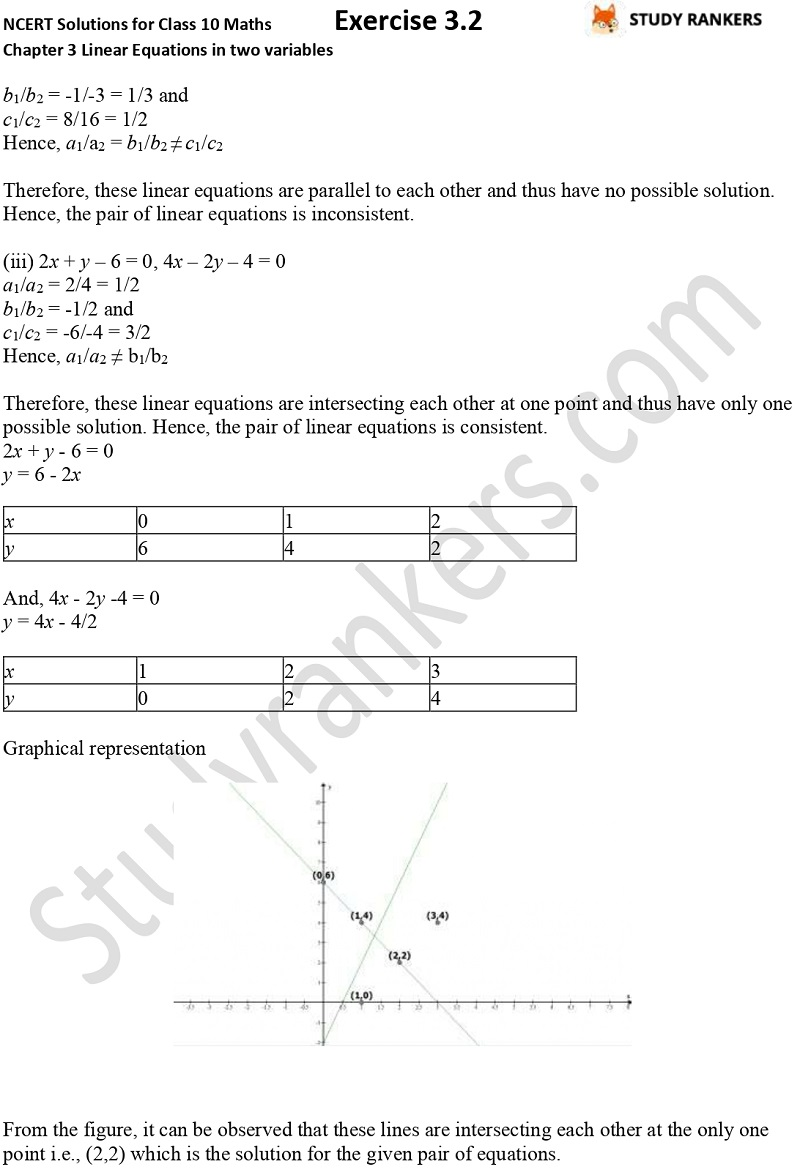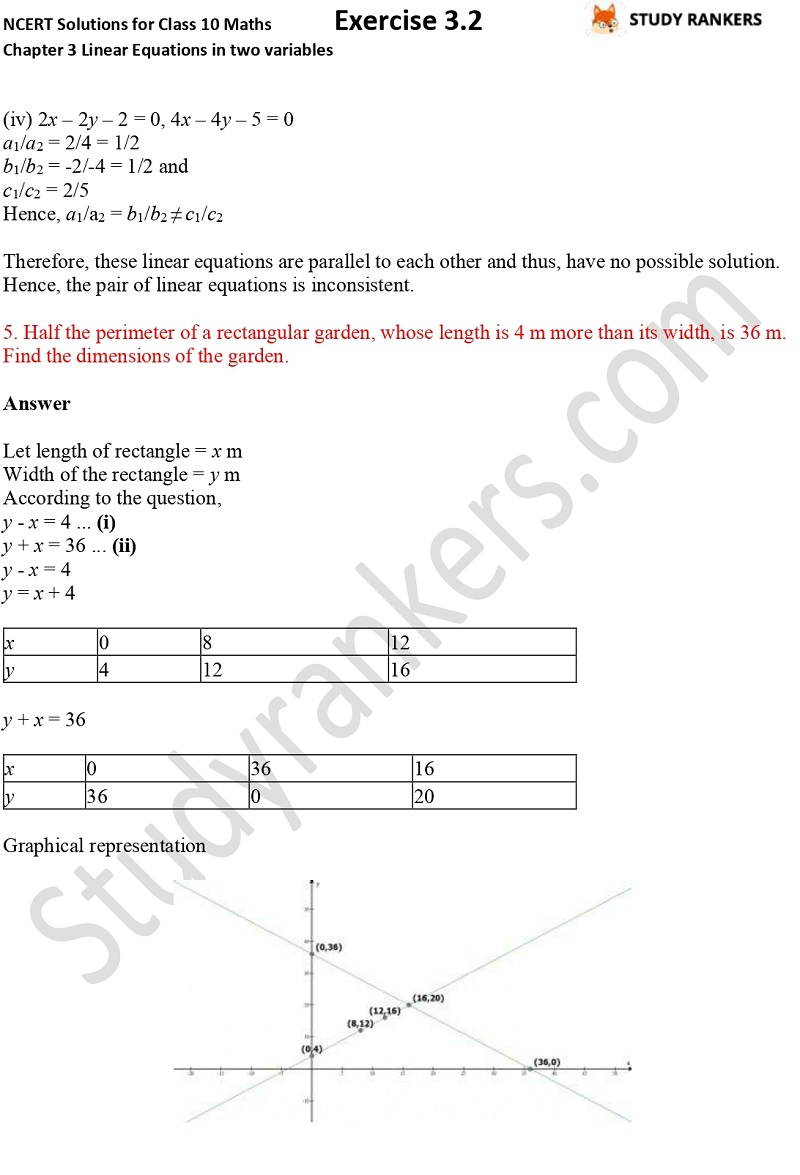## NCERT Solutions for Class 10 Maths Chapter 3 Pair of Linear Equations in Two Variables Exercise 3.2

We have provided Chapter 3 Pair of Linear Equations in Two Variables Exercise 3.2 Class 10 Maths NCERT Solutions here. It will help you in completing your homework if you're finding any problem difficult. Class 10 Maths NCERT Solutions will also introduce you to the concepts which can be used to solve problems. These solutions are updated according to the latest NCERT Maths textbook 2019-20. There are total seven questions in the exercise in which most of are word problems such as 5 pencils and 7 pens together cost Rs 50, whereas 7 pencils and 5 pens together cost Rs 46. Find the cost of one pencil and that of one pen.